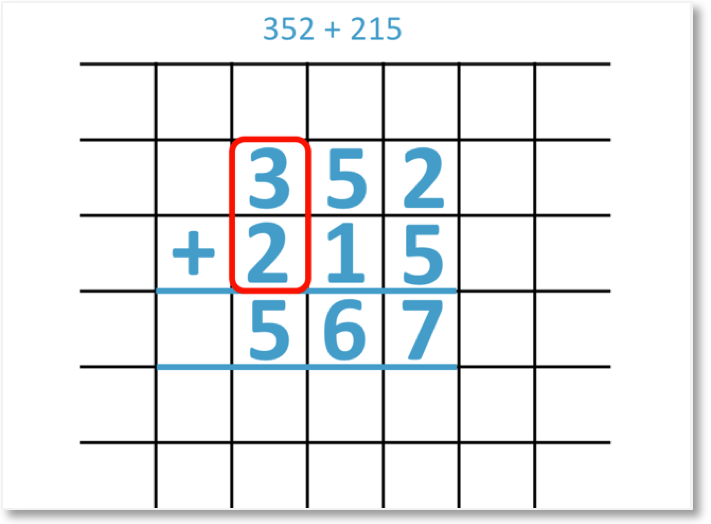# How to do Column Addition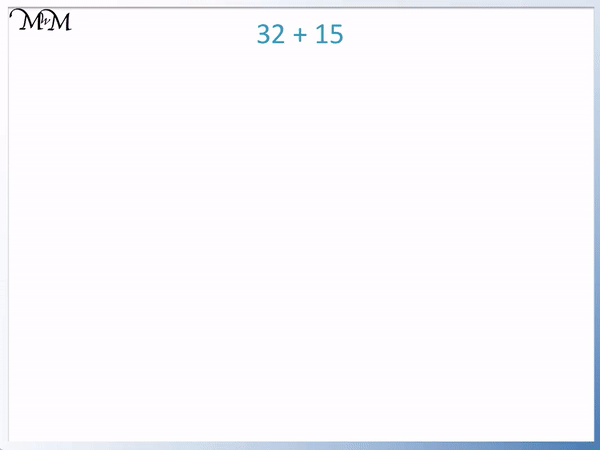• To add 32 and 15 we line up the individual digits in the tens and ones columns.
• We start on the right by adding the ones together.
• 2 + 5 = 7.
• We now add the digits in the tens column.
• 3 + 1 = 4.
• 32 + 15 = 47.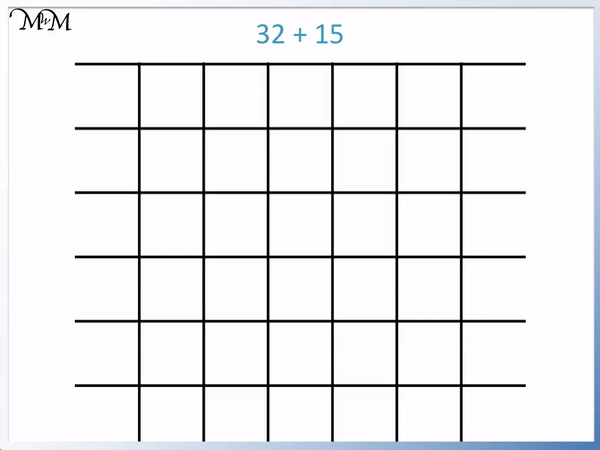Line up the digits above each other.

Add the digits separately from right to left.• To add 38 and 16 we separate the numbers into their tens and units.
• We start on the right by adding the units together.
• 8 + 6 = 14.
• We only write one digit per column in our answer.
• We regroup the 14 units into 1 lot of 10 and 4 units. We carry the 1 ten over to the tens column.
• There are 4 units remaining.
• We can now count number of groups of ten that we have in total.
• We have 3 plus 1, plus the extra 1 ten that we carried, which makes 5.
• 5 tens and 4 units makes 54 in total.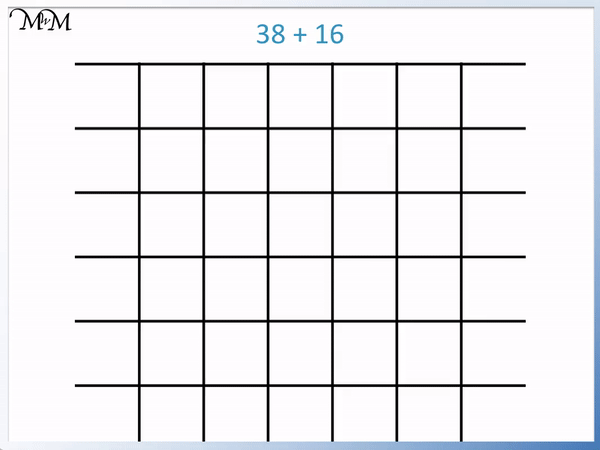Whenever the digits add to make 10 or more, carry the tens over to the tens column.• We line up the digits above each other and add them from right to left.
• 7 + 8 = 15.
• From the 15, we carry the 1 ten and write down 5 in the ones column.
• 2 + 4 + the 1 we carried = 7.
• 27 + 48 = 75.#### 2-Digit Column Addition: Interactive Question Generator

Column addition is the most commonly used written method for adding two or more numbers. The numbers are written with the digits lined up above each other to make columns. The digits in each column are added one at a time from right to left with the answer written below.

## How to Set Out Column Addition

1. Write the numbers above each other, lining up the digits in each place value column.
2. Draw 2 horizontal lines underneath these numbers to create the answer space.
3. Add the digits in each separate column starting with the rightmost column and finishing with the leftmost column.
4. Only write the ones digit of your answer below each column.
5. Write the tens digit of your answer below the answer space of the next column to the left.

It helps to use square grid paper when doing column addition because you can line up the digits of each number more carefully by writing each digit in each grid.

For example here is the column addition of 32 + 15.We line up the digits of each number according to their place value columns.

We work from right to left. So, we begin by adding the digits in the units column.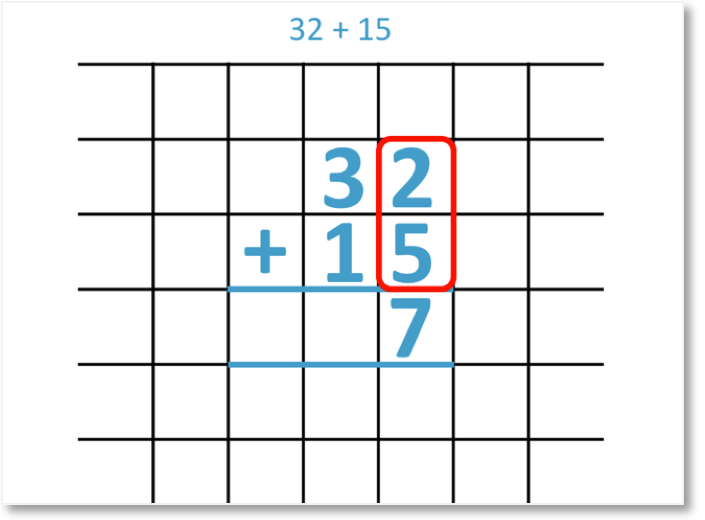2 + 5 = 7

We write our answer in the units column, underneath the 2 and the 5.

Next, we add the digits in the tens column.3 + 1 = 4

We write the answer in the tens column, underneath the 3 and the 1.

Therefore,

32 + 15 = 47

Here is the complete example showing the written column addition method as an animation.To add numbers without regrouping, firstly line up the digits of each number above each other. Add each column of digits separately. Write the answer to the addition below each column.

Regrouping is needed when the sum of digits in each column makes 10 or more. Addition without regrouping is when the digits in each column we add make an answer that is less than 10. This means that we can simply add the digits column by column and write the answer directly below.

For example here is 74 + 24.

We set out the addition like so, with the digits lined up above each other. It can be helpful to use square grid paper to assist with this layout.We start at the right and add the digits.

4 + 4 = 8

7 + 2 = 9

And so, 74 + 24 = 98.

Here is another example of column addition without regrouping.

In this example we want to add 53 + 31.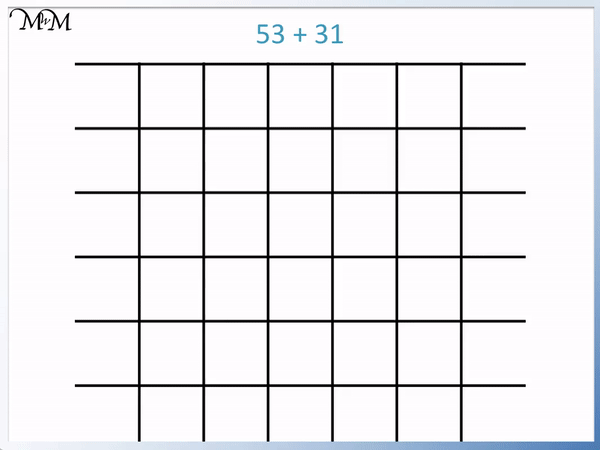Starting by adding the digits in the units column:

3 + 1 = 4

Then moving to the tens column:

5 + 3 = 8

And so, 53 + 31 = 84.

Column addition with regrouping occurs when the sum of any column is 10 or more. We only write down the units digit and carry the tens digit over to add to the next column on the left.

The rule of column addition is that we only write one digit in each answer box.

In regrouping questions, there are too many digits in the answer space for each place value column.

For example, here is 38 + 16.The number 38 has 8 units, which are shown by the eight counters in the units column.

It also has 3 tens, which are shown by the three groups of ten counters in the tens column.The number 16 has 6 units, which are shown by the six green counters in the units column.

It also has 1 ten, which is shown by the one group of ten green counters in the tens column.

First we, line up the digits of each number in their place value columns. Then we add the digits in each column.We have a total of 14 units in the units column. The number 14 has two digits.

We can’t write more than one digit in each place value column.

We therefore need to regroup some counters to carry over to the tens column.In the tens column, we count the groups of ten.

Therefore, we can regroup ten counters from the units column and carry them over to the tens column.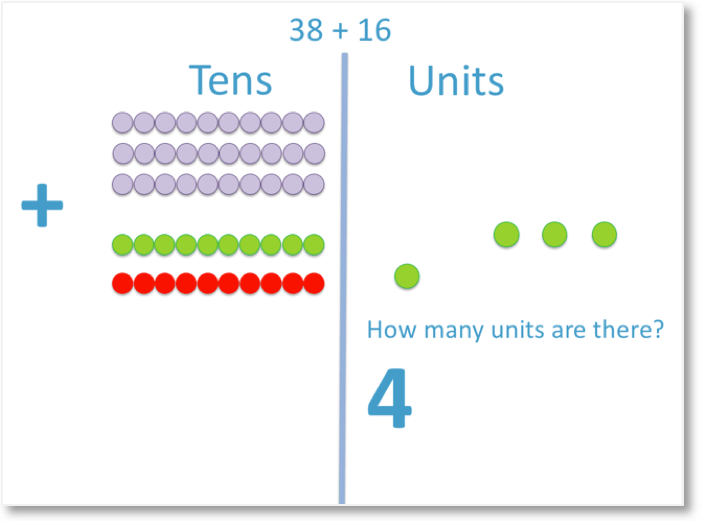Now that we have carried 1 lot of ten over to the tens column, we are left with 4 units in the units column.Altogether, there are 5 tens in the tens column, including the ten that we regrouped and carried from the units column.

Therefore,

38 + 16 = 54When the digits add to a number that is 10 or greater, it is necessary to do the regrouping process.

The ‘1’ is carried over to the next place value column and included with those numbers when the tens are added.

This process is called regrouping or carrying.

We’ll look at this example again, but this time we will use the formal written addition process.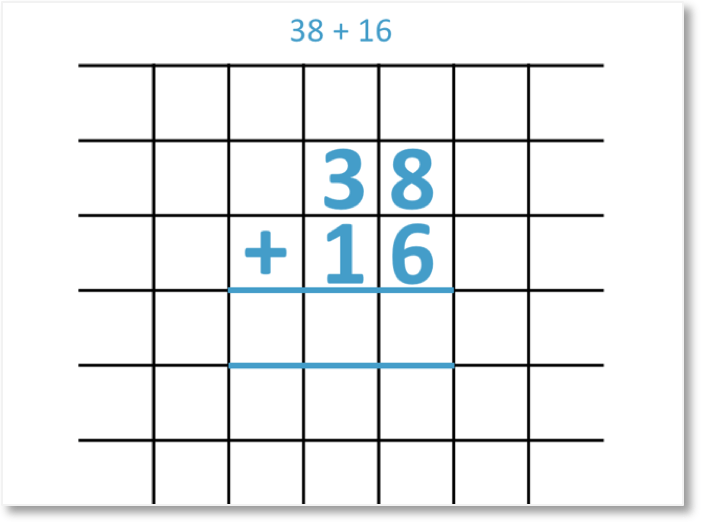First, line the digits up according to their place value columns.

We add columns from right to left. So, we begin with adding the digits in the units column.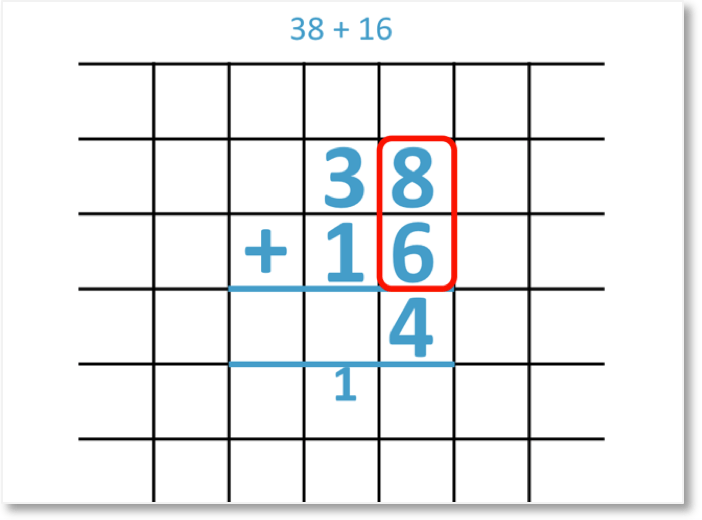8 + 6 = 14

The number 14 has 4 units, so we write 4 in the units column.

It also has 1 ten, so we carry ten over to the tens column and write it below the line.

Next, we add the digits in the tens column. We must also add the 1 ten that we carried over to the tens column.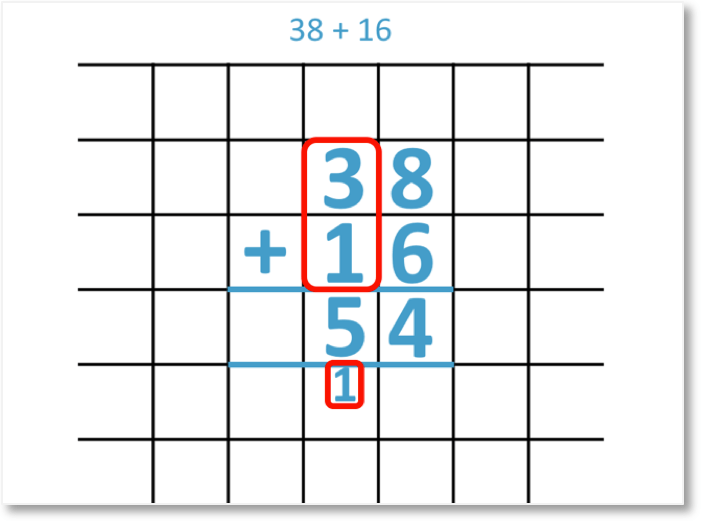3 + 1 + 1 = 5

Therefore,

38 + 16 = 54

Here is the complete method for adding the numbers shown on paper.It is best to teach the column addition process without carrying before introducing problems involving carrying.

## How to do Column Addition

1. Write the numbers directly above each other so that the digits in each place value column are lined up.
2. Add the digits in the ones column.
3. If this sum is 10 or greater, only write the ones digit of the answer and write the tens digit below the answer lines in the next column on the left.
4. Add the digits in the next place value column to any tens digits that you carried over from the previous step.
5. Repeat steps 3 and 4 until all place value columns have been added.
1. For example, 47 + 25.

Step 1 is to write the numbers directly above each other, lining up the digits.

We write the 7 above the 5 and the 4 above the 2.Step 2 is to add the digits in the ones column.

7 + 5 = 12

Step 3 is to write the ones digit of the answer and write the tens digit of the answer below the lines in the next column along.

12 contains two digits and so the ‘2’ digit is written and the ‘1’ is carried over to the tens column.

Step 4 is to add the digits in the tens column to any digits that were carried over in the previous step.

In the tens column we have 4 + 2.

We also have the ‘1’ ten that we carried over from our previous answer of 12.

4 + 2 + 1 = 7.

And so, 47 + 25 = 75.

It is a common mistake to forget to add the ‘1’ that has been carried and this is the first place to look for mistakes in column addition if the original procedure without carrying has been mastered.

The next example of column addition with carrying is 34 + 59.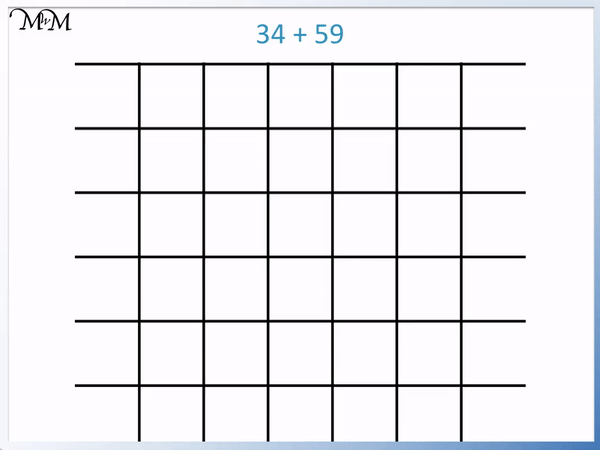Adding the digits in the units column we have:

4 + 9 = 13

We need to carry the ‘1’ over to the tens column and simply write ‘3’ in the units column.

Adding the digits in the tens column, we need to remember to add the ‘1’ that has been carried over.

3 + 5 + 1 = 9

Step 5 is to repeat steps 3 and 4 until all columns have been added.

34 + 59 = 93.

Here is another example of column addition with carrying.

We have 27 + 48.7 + 8 = 15.

We write down the ‘5’ and carry the ‘1’.

We need to add this ‘1’ to the numbers in the tens column.

2 + 4 + 1 = 7

And so, 27 + 48 = 75.

Column addition is taught in primary schools as the most common method for adding numbers. Lots of practice is recommended with using this written method. It helps to use counters or base ten blocks to introduce the idea of regrouping.

To add numbers using the column addition method we line the digits up in their place value columns and add the digits from right to left.

In the column addition example below, we are asked to calculate 32 + 15. We begin by partitioning the digits into their tens and units.

When first introducing the column addition method, it can be helpful to use counters to visualise the sizes of the numbers being added and to explain the carrying / regrouping process.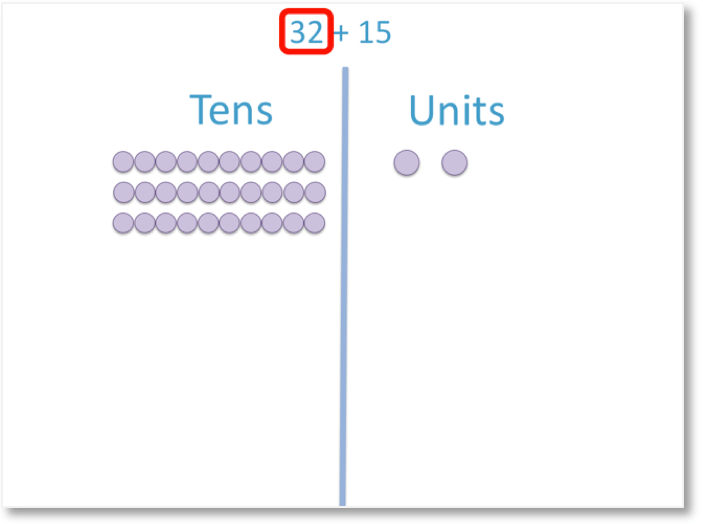The number 32 has 2 units. These are shown by the two counters in the units column.

It also has 3 tens. These are shown by the three groups of ten counters in the tens column.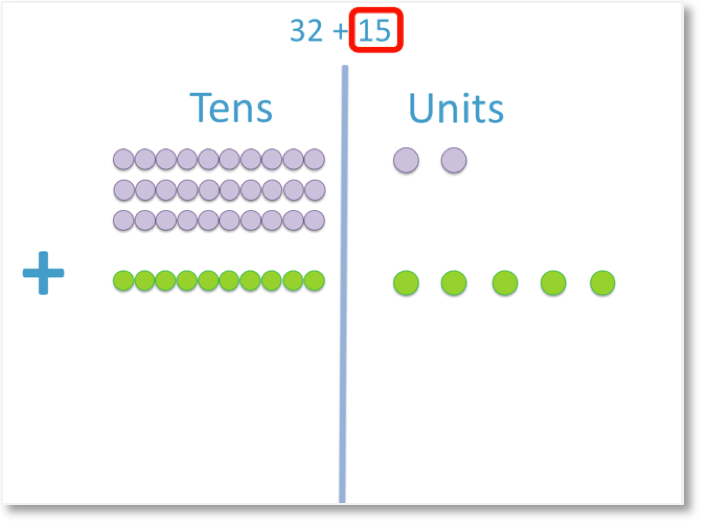The number 15 has 5 units. These are shown by the five green counters in the units column.

It also has 1 ten. This is shown by the one group of ten counters in the tens column.

Now that we have lined up the digits of each number in their place value columns, we can add the digits in each column.Altogether, in the units column, we have the 2 counters from the 32 and the 5 counters from the 15.

2 + 5 = 7

This gives us a total of 7 counters.Altogether, in the tens column, we have 3 groups of ten counters from the 32 and 1 group of ten counters from the 15.

3 + 1 = 4

This gives us a total of four groups of ten counters.

Therefore

32 + 15 = 47

Here is the full animated example.Now try our lesson on 3-Digit Column Addition without Carrying / Regrouping where we learn how to add three-digit numbers using column addition.